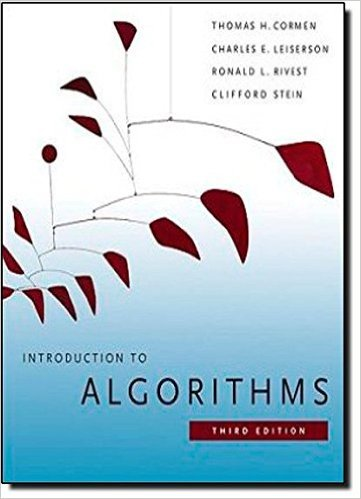×
Get Full Access to Introduction To Algorithms - 3 Edition - Chapter 22 - Problem 22-1
Get Full Access to Introduction To Algorithms - 3 Edition - Chapter 22 - Problem 22-1

×

# Classifying edges by breadth-first search A depth-firstISBN: 9780262033848 130

## Solution for problem 22-1 Chapter 22

Introduction to Algorithms | 3rd Edition

• Textbook Solutions
• 2901 Step-by-step solutions solved by professors and subject experts
• Get 24/7 help from StudySoup virtual teaching assistantsIntroduction to Algorithms | 3rd Edition

4 5 1 432 Reviews
21
4
Problem 22-1

Classifying edges by breadth-first search A depth-first forest classifies the edges of a graph into tree, back, forward, and cross edges. A breadth-first tree can also be used to classify the edges reachable from the source of the search into the same four categories. a. Prove that in a breadth-first search of an undirected graph, the following properties hold: 1. There are no back edges and no forward edges. 2. For each tree edge .u; /, we have :d D u:d C 1. 3. For each cross edge .u; /, we have :d D u:d or :d D u:d C 1. b. Prove that in a breadth-first search of a directed graph, the following properties hold: 1. There are no forward edges. 2. For each tree edge .u; /, we have :d D u:d C 1. 3. For each cross edge .u; /, we have :d u:d C 1. 4. For each back edge .u; /, we have 0 :d u:d.

Step-by-Step Solution:
Step 1 of 3

\ f . 4"L '\ I I ,J2{ \ ;y t VII {-) -&'/ I -.rdplB \l Uo q/.n\Aler)h.rn fl )-fl".ipat.fi)ta\) q \ Uq-up\Jrn3 \_/ Zrre- Lt,.l \ I- "\- i h: h-) Y cl.-v ,t \"1*'\ = -\*./J -V \:a1,1-'l -T'l--- tu'\l -\A ,c

Step 2 of 3

Step 3 of 3

##### ISBN: 9780262033848

The full step-by-step solution to problem: 22-1 from chapter: 22 was answered by , our top Engineering and Tech solution expert on 11/10/17, 05:55PM. Since the solution to 22-1 from 22 chapter was answered, more than 245 students have viewed the full step-by-step answer. This textbook survival guide was created for the textbook: Introduction to Algorithms, edition: 3. This full solution covers the following key subjects: edges, edge, breadth, search, tree. This expansive textbook survival guide covers 35 chapters, and 151 solutions. The answer to “Classifying edges by breadth-first search A depth-first forest classifies the edges of a graph into tree, back, forward, and cross edges. A breadth-first tree can also be used to classify the edges reachable from the source of the search into the same four categories. a. Prove that in a breadth-first search of an undirected graph, the following properties hold: 1. There are no back edges and no forward edges. 2. For each tree edge .u; /, we have :d D u:d C 1. 3. For each cross edge .u; /, we have :d D u:d or :d D u:d C 1. b. Prove that in a breadth-first search of a directed graph, the following properties hold: 1. There are no forward edges. 2. For each tree edge .u; /, we have :d D u:d C 1. 3. For each cross edge .u; /, we have :d u:d C 1. 4. For each back edge .u; /, we have 0 :d u:d.” is broken down into a number of easy to follow steps, and 161 words. Introduction to Algorithms was written by and is associated to the ISBN: 9780262033848.

Unlock Textbook Solution